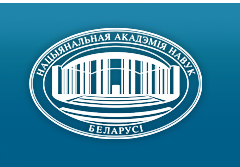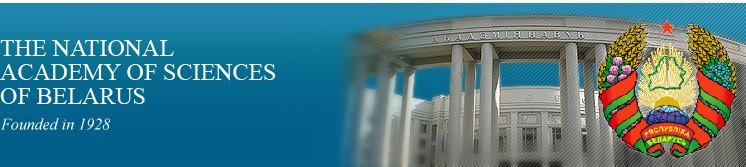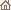Home News About Academy Academy Activities Academy Membership Organizations Publications Conferences Contacts Site Map

# Proc. of the Natl. Academy of Sciences of Belarus, Ser. Phys.-Math. Sci., 1999, No.1/ Publications / Scientific Journals

Proc. of the Natl. Academy of Sciences of Belarus, Ser. Phys.-Math. Sci., 1999, No.1Proceedings of the National Academy of Sciences of Belarus (Vestsi Natsiyanalnai Akademii Navuk Belarusi) SERIES OF PHYSICAL-MATHEMATICAL SCIENCESPublished by The Belaruskaya Navuka Publishing House, Minsk, Republic of Belarus

### Number 1, 1999## CONTENTS

### MATHEMATICS

Garist Yu.Ye., Garist V.Ye., Palchik Ye.M.
About finite groups which admitted a cosimple automorphism with nilpotent stabilisator. pp. 5--7

Summary: The following proposition is proved. Let X be a finite solvable group admitting automorphism у of prime order r, (|X|, r) = 1 and В = Сx(y) is a nilpotent subgroup. Let be P = P (B) and p Î P. If p = 2 then suppose that r ¹ 2k + 1. Let P be a y-invariant Sp-subgroup of X. Then either [y, P] << X, or Bp << X.

Laptinsky V.N.
On theory of twoperiodic solutions of linear partial hyperbolic systems. pp. 8--12

Summary: The algorithm of the construction of two periodic solutions of linear partial hyperbolic systems is worked out.

Titov V.L.
Some representation of periodic solutions of quasilinear differential systems. pp. 13--17

Summary: Constructive conditions of the existence and uniqueness of periodic solution for semilinear ordinary differential system are obtained.

Radyno N.Ya.
About the solutions of a linear differential equations in the algebra of mnemofunctions containing tempered distributions. pp. 18--22

Summary: The definition and construction of the solution of a linear differential equation with a constant coefficients P(D)u = d in the algebra of mnemofunctions which contains the tempered distributions are proposed. Then we give the physical illustration of the results on the example of the equation Du = d is given.

Petrov V.A.
On approximate evaluation of Gaussian continual integrals by diagrammatic approach. pp. 23--27

Summary: Approximate evaluation of continual integrals on Gaussian measure on the basis of diagrammatic method are considered. The integrable functional is a function of quadratic functional of special form.

Demenchuk A.K.
On quasiperiodic solutions of differential systems with multifrequency nonlinearity. pp. 28--30

Summary: The existence of quasiperiodical solutions of ordinary differential system is reduced to analogous existence problem for smaller dimention system.

Manyuk E.M., Yanovich L.A.
On some interpolation formulas for operators in functional spaces. pp. 31--36

Summary: The interpolation formulas for operators based on Lagrange and Hermit interpolation polynomials are constructed.

Gusarevich L.D.
On the integral equation with two kernels solvable by the Kontorovich-Lebedev transform. pp. 37--44

Summary: An integral equation with two convolutions is investigated. It can be reduced to the Riemann boundary value problem by means of the Kontorovich-Lebedev transform. One of the convolution is the Kontorovich-Lebedev. Necessary properties of them are established. The connection between Cauchy's type integral and the Kontorovich-Lebedev integral is obtained.

Mukhin V.V., Boujouf Hamza
The topological n-ary semigroup. pp. 45--48

Summary: Conditions on the topology of the topological n-ary semigroup is established. The topology defined on the topological n-ary semigroup can be extended to the topology compatible with the semigroup operation defined on the enveloping group.

Zhuk E.E.
About some method for cluster analysis of multivariate probability distribution mixture. pp. 49--55

Summary: The problem of cluster analysis of multivariate probability distribution mixture is considered. The new "plug-in" decision rule (PDR) based on estimation of unknown class characteristics (mixture parameters) by the generalized method of moments are proposed. As the efficiency measure of PDR a risk (classification error probability) is used and evaluated by the asymptotic expansion method. The obtained results are illustrated for the well known Fisher model which is often applied for the experimental data description on practice.

Gushchinskii N.N., Levin G.M.
Optimization of quasiadditive functions over a set of network of parameters. pp. 56--60

Summary: The problem to optimize parameters of network arcs is considered. The optimization function and constraints depend on length of all paths from source to sink and to all intermediate vertices of network. The system of invariants is investigated that allows to parameterize the original problem and to reduce it to the problem of network vertex parameter optimization. In order to solve the obtained problem the decomposition methods are proposed taking into account network topology.

### PHYSICS

Rubinov A.N., Efendiev T.Sh., Katarkevich V.M., Ryzhechkin S.A., Afanas'ev A.A.
Generation of ultrashort pulses in a holographic DFB dye laser with traveling-wave pumping. pp. 61--65

Summary: A numerical and experimental investigations are reported of the special features of generation of the holographic dye laser with distributed feedback (DBF) and pumped by a traveling wave. It is shown that using picosecond (3ps) "skew" pump pulses (pulses with non-coincident amplitude and phase fronts) makes it is possible to generate single (»0.8 ps) ultrashot pulses.

Ivanov A.P., Vitkin Ye.I.
Temperature and radiation field structure inside a heated dispersive slab. pp. 66--69

Summary: This investigation is a continuation of the work . It is has stated and solved the differential equation of radiation and heat transfer as well as evaluated the effects of optical and thermophysical parameters when a plane-parallel light-scattering slab transits to a steady state under heating by literal plates and internal nets. This work considers the temporal transformation of the temperature and radiation field over the slab depth as a function of the thermal conductivity, the number of nets and optical properties.

Korshunov F.P., Kurilovich N.F., Prokhorenko T.A., Bumai Yu.A., Ulyashin A.G.
The influence of g-irradiation Co60 on electrical properties of undoped GaAs treated with hydrogen plasma. pp. 70--76

Summary: The influence of exposition to a hydrogen plasma (hydrogenation) on the electrical properties alteration under g-irradiation in bulk GaAs have been investigated. It is shown that crystals hydrogenation before irradiation leads to particulary passivation of electrically active defects that are responsible for carriers scattering and removing processes in irradiated crystals. Radiation defects termostability in hydrogenezated GaAs crystals is lower than that in nonhydrogenezated ones. The energetic levels position of main defect that effects on electrical properties alteration after irradiation in GaAs crystals was detected. It is equal to ED = EC - 0.125 ± 0.0005 eV.

Pletyukhov M.V., Tolkachev E.A.
Sp(2)-covariant generalization of the Hurwitz transformation. pp. 77--80

Summary: The Sp(2)-matrix representation for the Hurwitz transformation has been constructed and the quaternion Hopf bundle has been generalized. Covariant expressions for SU(2)-instantonic Yang-Mills potential have been obtained.

Avakyan E.Z., Avakyan C.L.
On decompositions K+ ® p+ l+ l-. pp. 81--86

Summary: The rare K+ ® p+ l+ l- decays are studied by using the effective Hamiltonian of weak interaction. The contribution from intermediate mesons is shown to be important for description of above mentioned decays. The calculated branching ratio for K+ ® p+ e+ e- is in a god agreement with experimental data. The branching ratio for K+ ® p+ m+ m- is predicted: Br(K+ ® p+ m+ m-) = 0.73 ± 0.14 x 10-7.

Sandrigaylo L.E.
The influence of absorption on the radial distribution of temperature in electrical arc plasma. pp. 87--89

Summary: The results of calculation radial distribution temperature of electrical air arc with Cu, Ca, Na impurities and absorption are presented.

Savastenko N.A.
Formation of C2 molecules in laser-produced plasma. pp. 90--93

Summary: Optical emission studies of C2 molecules in plasma obtained by XeCl-laser ablation of graphite in a helium atmosphere are reported. The characteristics of the spectral emission intensity from C2 (Swan band) species have been investigated as function of distance from the target and ambient pressure. Oscillating temperatures of C2 species for various helium.pressure are estimated. The additional data have been received to confirm recombination mechanism for the production of C2 molecules.

Gubar N.B., Tolkacheva E.G.
Investigation of SRS process with account of anti-Stokes and backward Stokes components. pp. 94--99

Summary: In this work we made theoretical analysis of the SRS-process with account of anti-Stokes, forward and backward Stokes components. We investigated the influence of backward Stokes emission in pump depletion regime. Transverse structure of forward and backward Stokes components has been discussed in the given pump beam approximation.

Budkevich B.A., Ges I.A., Romanov I.M.
About possibility of application of the phenomenon of coloration centers transformation in tungsten oxide electronic films for detecting of hydrogen atoms. pp. 100--102

Summary: In work offered method of metallic palladium precipitation based on colour centers transformation in oxide tungsten film. The film of palladium possessed a developed surface and served the basis for hydrogen sensor of resistive type on the base of thin-film structure WO3--Pd. The basic characteristics of sensor are investigated. The received results testify to efficiency of an offered method of palladium precipitation when making sensitive layer of hydrogen sensors of resistive type.

Liopo V.A.
Hexagonal lattice in 4-dimensional space. pp. 103--106

Summary: The transitions between different crystal system are considered. The base matrix is determined as matrix with 0, ± 1 and number ±1 is only one in every rows-matrix ár| = ám, n...| or column-matrix |сñ = |p, q, ...ñ where m, n ...; p, q ... are the sign and number of nonzero elements in rows or in columns. There are base (hypercube) lattices isomorphic for 3-D nonbase and 3-D noncrystallographic symmetries. Hexagonal lattice in the 3-D projection of 4-D hypercube.

### INFORMATICS

Lipnitskii S.F., Shibut M.S., Yarmosh N.A.
Hypertext expert-tutorial. II. Algorithms of computer-assisted learning. pp. 107--111

Summary: Architecture of expert-tutorial system is presented. On the base of mathematical data and knowledge models algorithms of computer-assisted learning route generation are proposed.

Babaitsev A.Yu, Tyshkevich R.I.
Linear dimension of split graphs. pp. 112--115

Summary: A graph G can be represented as a union G = G1 È ... È Gk of pair wise edge-disjoint graphs Gi with the same vertex set VG such that all connected components of Gi are complete graphs, the smallest number k of graphs Gi, in such representation is called the linear dimension ld(G). It is proved that the linear dimension of a split graph can be computed with a linear complexity. Moreover, for any constant k the split graphs with ld(G) £ k can be characterized by means of a finite list of forbidden.

Zverovich I.Ye.
The existence of a finite forbidden induced subgraph characterization for an arbitrary hereditary class of l-densed graphs. pp. 116--118

Summary: A hereditary class P is called l-densed, l ³ 0 it w(G) ³ |VG| - l where w(G) is the clique number of G. It is shown that every l-densed class has a finite forbidden induced subgraph characterization. This result is applied to the line graphs of linear k-uniform hypergraphs.

Malinkovskii Yu.V., Yakubovich O.V.
Closed queuing networks with the rounds of the nodes by customers. pp. 119--124

Summary: It is considered a closed network with M customers. It is assumed that isolated node is described by the regular Markov chain. Customer being sent to the node joines it with a probability depends on a state of the node or rounds it with the complementary probability. It is proved that boundly M-quasireversibility in view of rounds is a sufficient condition for product form of stationery distribution.

Gagarin A.V.
Line graphs of topologicai hereditary classes. pp. 125--129

Summary: Topological hereditary classes are studied. We give a solution of the following problem: find out the set Y of forbidden induced subgraphs for the class of line graphs L(P) by the known set X of forbidden topological subgraphs for a topological hereditary class P. A method to construct the set Y is proposed. As a result of the research, we characterize the line graphs of planar graphs in terms of forbidden induced subgraphs.

Chernyak A.A.
Algorithmic complexity of graph reliability problems. pp. 130--135

Summary: It is known that the problem of computing the all-terminal reliability is #P-complete for planar graphs while being polynomially solvable for trees, series-parallel graphs and complete graphs. In this paper it is proved that the problem of computing all-terminal reliability is #P-complete for bipartite graphs of arbitrary girth and for split graphs with the regular part of degree 2.

### ABSTRACTS OF DEPOSITED PAPERS

Minchenko L.I., Satsura T.V.
Topological and differential properties of optimal solutions in mathematical programming problems with perturbation. p. 136

Zaika V.A.
Amplitude-temporal selection during long duration experimental data gathering. p. 136

Zaika V.A., Lopareva N.V., Vankovich G.R., Klimentovsky V.V.
Modular conversion of contiguous data flows in of full-scale experiments and tests automotion systems. p. 137

Pavlov L.I.
Photoelectric device for the measuring of the mutual displacement of two systems of interferential strips in Kesters interferometer. p. 137

Petrov P.K.
Variational superposition of boundary value in the problems of light diffraction on solids with complex forms. pp. 137--138/ Publications / Scientific Journals / Back to Top of Page

Designed and maintained by Dr. Nikolai N. Kostyukovich. Last updated: May 14, 1999
Created with assistance of Dr. Ignatii I. Korsak
Copyright © 1999 The National Academy of Sciences of Belarus
Copyright © 1999 The Belaruskaya Navuka Publishing House